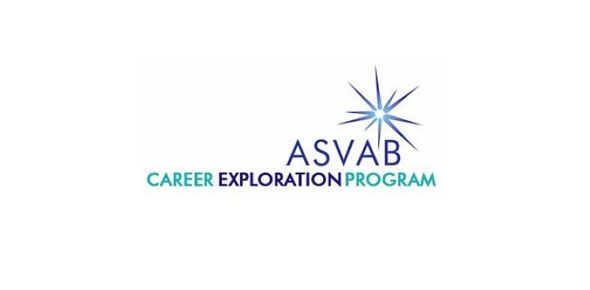# Hunters Homemade ASVAB Test - Wordknowledge/Electronicsinfo - Multiple Choice - 30 Questions

31 Questions | Attempts: 159
ShareSettings• 1.
Exempt most nearly means
• A.

Empty

• B.

Exit

• C.

Excuse

• D.

Anoint

• 2.
The decision was unanimous
• A.

Questioned

• B.

Undisputed

• C.

Selective

• D.

Trivial

• 3.
Temperamental most nearly means
• A.

Benevolent

• B.

Lazy

• C.

Volatile

• D.

Demure

• 4.
Mark was more conscientious than his friends
• A.

Painstaking

• B.

Popular

• C.

Friendly

• D.

Handsome

• 5.
Profess most nearly means
• A.

Work

• B.

Claim

• C.

Disguise

• D.

Accuse

• 6.
The substance of their argument was trivial.
• A.

Sinister

• B.

Inconsequential

• C.

• D.

Demanding

• 7.
The view was panoramic.
• A.

Beautiful

• B.

Unrealistic

• C.

Stylistic

• D.

Expansive

• 8.
Contingent most nearly means
• A.

Rapid

• B.

Dependent

• C.

Difficult

• D.

Mysterious

• 9.
Velocity most nearly means
• A.

Variety

• B.

Veracity

• C.

Swiftness

• D.

Overflow

• 10.
The wind was so strong that the boat capsized.
• A.

Overturned

• B.

Accelerated

• C.

Tilted

• D.

Docked

• 11.
The elected official abdicated responsibility for the situation.
• A.

Welcomed

• B.

Cheered

• C.

Transistioned

• D.

Abandoned

• 12.
Her skills made her eligible for the position.
• A.

Qualified

• B.

Eager

• C.

• D.

Thankful

• 13.
Meander most nearly means
• A.

Twist

• B.

Reverse

• C.

Exceed

• D.

Renew

• 14.
Infiltrate most nearly means
• A.

Interfere

• B.

Seek

• C.

Enter

• D.

Penetrate

• 15.
His science grade plummeted after that difficult exam.
• A.

Soared

• B.

Plunged

• C.

Increased

• D.

Failed

• 16.
One hertz is defined as
• A.

One ampere per second

• B.

One cycle per second

• C.

One volt per second

• D.

One coulomb per second

• 17.
One advantage of alternating current is that it is easy to
• A.

Store

• B.

Convert into chemical energy

• C.

Transport through wire

• D.

Transmit through air

• 18.
Electric current can travel only through a
• A.

Wire

• B.

Circuit

• C.

Battery

• D.

• 19.
Copper is used in most electric wires because it is
• A.

Aa semiconductor

• B.

Not likely to heat up during normal usage.

• C.

A good resistor and inexpensive.

• D.

A good conductor and inexpensive.

• 20.
Amperes area measure of
• A.

The number of electrons moving through a conductor

• B.

Electrical pressure

• C.

A materials ability to store electric current

• D.

The total resistance of a series circuit

• 21.
Ohms are a measure of
• A.

Capacitance

• B.

Resistance

• C.

Current

• D.

Cycles per second

• 22.
A circuit with 20 amperes has a load of 12 ohms. what is the voltage?
• A.

60

• B.

120

• C.

240

• D.

24

• 23.
If the resistance of a circuit is 0, the circuit is
• A.

An open circuit

• B.

A short circuit

• C.

A superconductor circuit

• D.

A semiconductor circuit

• 24.
If a transformer raises the voltage, it will
• A.

Raise the resistance

• B.

Reduce the resistance

• C.

Raise the amperage

• D.

Reduce the amperage

• 25.
Which of these devices depends on the close relationship between the electricity and magnetism?
• A.

Transformer

• B.

Electromagnet

• C.

Electric motor

• D.

All of the above

## Related TopicsBack to top
×

Wait!
Here's an interesting quiz for you.